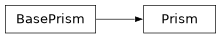# geoana.gravity.Prism#class geoana.gravity.Prism(min_location, max_location, rho=1.0)#

Class for gravitational solutions for a prism.

The Prism class is used to analytically compute the gravitational potentials, fields, and gradients for a prism with constant denisty.

Parameters
min_location(3,) array_like

(x, y, z) triplet of the minimum locations in each dimension

max_location(3,) array_like

(x, y, z) triplet of the maximum locations in each dimension

rhofloat, optional

Density of prism ($$\frac{kg}{m^3}$$).

Attributes

 location The center of the prism mass The mass of the prism max_location Location of the point mass. min_location Location of the point mass. rho The density of the prism. volume The volume of the prism

Methods

 Gravitational field due to a prism. Gravitational gradient due to a prism. Gravitational potential due to a prism.